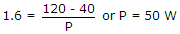# Electronics and Communication Engineering - Power Electronics

### Exercise :: Power Electronics - Section 1

6.

A semiconverter feeding an RLE load operates in

Explanation:

Semiconvertor operates only in first quadrant.

7.

The phenomenon of overlap in converter operation due to

 A. source resistance B. source inductance C. both source resistance and source inductance D. high value of firing angle

Explanation:

Source inductance delays turn off and hence causes overlap.

8.

A thyristor has a maximum allowable junction temperature of 120°C and the ambient temperature is 40°C. If thermal resistance is 1.6° C/W, the maximum allowable internal power dissipation is

 A. 20 W B. 50 W C. 92 W D. 128 W

Explanation:.

9.

For a 12 pulse operation, the two 6 pulse units are fed by

 A. Y - Y and Y - Y transformers B. Δ - Δ and Δ - Δ transformers C. Y - Y and Y - Δ transformers D. Y - Δ and Y - Δ transformers

Explanation:

30° phase difference is necessary.

10.

In a single phase full wave converter (M - 2 connection) feeding a highly inductive load, the firing angle for each thyristor is a in the respective half cycle. The period of conduction of each thyristor is

 A. p - a B. p C. p + a D. p - 2a